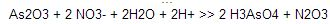# As2o3(s)+no−3(aq)→h3aso4(aq)+n2o3(aq) (acidic solution)

Complete and balance the foloowing equation: As2O3(s)+NO3^-(aq)—->H3AsO4(aq)+N2O3(aq) (acidic
solution)

b) As2O3 + 5H2O >> 2 H3AsO4 + 4H+ + 4e-
2 NO3- + 6H+ + 4e- >> N2O3 + 3H2O
————————————–…
As2O3 + 2 NO3- + 2H2O + 2H+ >> 2 H3AsO4 + N2O3
As goes from +3 to +5
N goes from +5 to +3
or
As2O3 > 2 H3AsO4 & 4 e- lost …….(2 As+3 each –> 2
As+5 each)
2 (NO3)-1 & & 4 e- taken > N2O3 … (2 N+5 each –> 2
N+3 each)
since they are balanced in electrons taken & lost, combine
them:
1As2O3(s) + 2NO3-(aq) > 2H3AsO4 (aq) + N2O3 (aq)
the imbalances are:
zero H’s <-> 6 H’s
9 oxygens <-> 11 oxygens
-2 ion charge <-> no charge
I will be able to later balance the need on the left for H’s &
O’s with neutral H2O later, so I am going to balance the charges
first, by adding 2 negative hydroxides to the right side:
As2O3(s) + 2NO3-(aq) > 2H3AsO4 (aq) + N2O3 (aq) & 2
OH-
with the charges now balanced, I notice that I now have an excess
of 8 Hydrogens & 4 oxygens on the right , which can be balanced
by adding 4 H2O’s to the left:
1As2O3(s) + 2NO3-(aq) & 4 H2O > 2H3AsO4 (aq) + 1N2O3 (aq)
& 2 OH-
As2O3 + 2 NO3- + 2H2O + 2H+ ——–>> 2 H3AsO4 +
N2O3
As goes from +3 to +5
N goes from +5 to +3